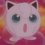# Cosine trouble

Recently I was studying work and energy chapter, i saw the formula for 'work done when a body moves at an angle in the direction of force, it was given as -

                       Work done = Force cos θ * distance moved by the body


The book is saying that according to the table of natural cosines....cos 60 deg = 0.5 But in exams we can't look up at the table or don't get time to draw any equilateral triangle etc, to calculate cosine of any angle, please help, isn't there any formula to take out the cosine of any angle, there must be one, because by the formula only the cosine of different angles must have been found out, and then the table must have been prepared. Please help and share your views.Note by Sahba Hasan
7 years, 2 months ago

This discussion board is a place to discuss our Daily Challenges and the math and science related to those challenges. Explanations are more than just a solution — they should explain the steps and thinking strategies that you used to obtain the solution. Comments should further the discussion of math and science.

When posting on Brilliant:

• Use the emojis to react to an explanation, whether you're congratulating a job well done , or just really confused .
• Ask specific questions about the challenge or the steps in somebody's explanation. Well-posed questions can add a lot to the discussion, but posting "I don't understand!" doesn't help anyone.
• Try to contribute something new to the discussion, whether it is an extension, generalization or other idea related to the challenge.

MarkdownAppears as
*italics* or _italics_ italics
**bold** or __bold__ bold
- bulleted- list
• bulleted
• list
1. numbered2. list
1. numbered
2. list
Note: you must add a full line of space before and after lists for them to show up correctly
paragraph 1paragraph 2

paragraph 1

paragraph 2

[example link](https://brilliant.org)example link
> This is a quote
This is a quote
    # I indented these lines
# 4 spaces, and now they show
# up as a code block.

print "hello world"
# I indented these lines
# 4 spaces, and now they show
# up as a code block.

print "hello world"
MathAppears as
Remember to wrap math in $$ ... $$ or $ ... $ to ensure proper formatting.
2 \times 3 $2 \times 3$
2^{34} $2^{34}$
a_{i-1} $a_{i-1}$
\frac{2}{3} $\frac{2}{3}$
\sqrt{2} $\sqrt{2}$
\sum_{i=1}^3 $\sum_{i=1}^3$
\sin \theta $\sin \theta$
\boxed{123} $\boxed{123}$

Sort by:

Sadly, you'll have to memorize the values of cosine. Thankfully, they mostly ask the cosines of five angles, $0^\circ,30^\circ,45^\circ,60^\circ,90^\circ$

You can use a table to memorize those.

 $\cos 0^\circ$ $\cos 30^\circ$ $\cos 45^\circ$ $\cos 60^\circ$ $\cos 90^\circ$ $\sqrt{\frac{4}{4}}$ $\sqrt{\frac{3}{4}}$ $\sqrt{\frac{2}{4}}$ $\sqrt{\frac{1}{4}}$ $\sqrt{\frac{0}{4}}$

- 7 years, 2 months ago

Thank you, but that's sad that i have to memorize, but then how was this table created?

- 7 years, 2 months ago

Yes, you would have to learn the cosines, sines and tans of mostly 5 angles i.e. 0, 30 , 45, 60, 90

- 6 years, 9 months ago